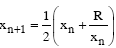# GATE | GATE CS 2008 | Question 85

• Last Updated : 28 Jun, 2021

The Newton-Raphson iterationcan be used to compute the.
(A) square of R
(B) reciprocal of R
(C) square root of R
(D) logarithm of R

Explanation: According to Newton-Raphson method,

`xn+1 = xn − f(xn) / f′(xn) `

So we try to bring given equation in above form. Given equation is :

```xn+1 = xn/2 + R/(2xn)
= xn − xn/2 +  R/(2xn)
= xn − (xn2 − R2)/(2xn) ```

So clearly f(x) = x2 − R, so root of f(x) means x2 − R = 0 i.e. we are trying to find square root of R. So option (C) is correct.

My Personal Notes arrow_drop_up